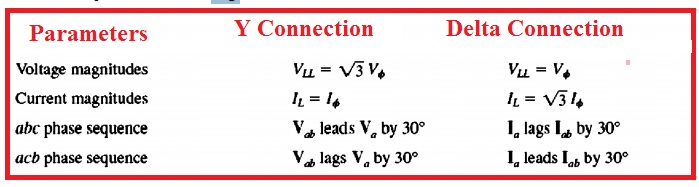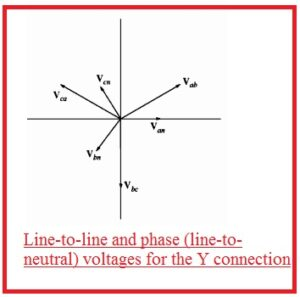The main benefit to use this system is that as compared to the dc system in AC three-phase system the voltage value can be changed according to the required load at any instant through the use of a transformer.

As compared to the single-phase system there is 2 main benefit over the single-phase system that large power can deliver through this system and power delivered through this system remain constant all over the transmission that lack in case of the single phase where it varied. In this post, we discuss its types of working and some other related parameters. So let get started with

How Three Phase Current and Voltages Generates

• In the three-phase generator, there is 3 single phases generator are assembled that offered the same value of voltage having one twenty-degree out of phase condition.• This configuration of generators makes 3 single-phase circuit arrangments that are configured in 120 degrees apart.
• The current equation for every circuitry is mentioned here.

I=V/Z

• Current flowing in the 3 phase circuits are given here.• it is obvious to make link at the negative points of 2 single-phase generators to get the common line or neutral.
• In the below figure we can see this arrangement.• From this, we can observe that there is 4 wires are used in the three generators system to deliver power to the load.
• The current flowing In the neutral wire is the sum of other three wire current and mentioned here.• there is similar values of 3 loads so there is will be 0 return current flow in the neutral common wire.

### What is a balance three-phase system

• Such an electrical system that has same values of voltage for 3 generators is same and one twenty degrees out of phase called balance three-phase system.

What is phase sequence?

• The phase sequence is the sequence of 3 phase network that voltage in single phases are arranged.
• The 3 phase power system is explained here.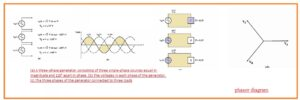• There is phase sequence for this system is abc and their order is a b c.
• The phasor diagram of the system having abc phase sequence can see here.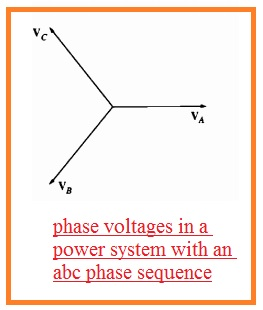• It is certain to link 3 phases of power to get the phase order a, c, b in this system phase sequence is acb
• The phasor diagram of the system acb phase can be seen here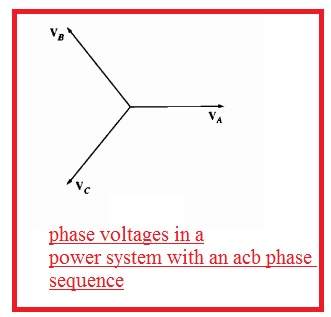• The outcome is used for these 2 phase sequences abc and acb.
• In these two system, the power is balanced and zero current flow in the neutral

### Three Phase Circuits Voltage and Current

• The connection configuration in the circuit shown is called wye due to Y-like arrangements.• There is another connection configuration in which generators are linked from head to tail arrangements and called delta connection.
• In delta connection, there is zero value of voltage among the three-phase VA+VB+VC=0
• In this connection arrangement, there is zero fault current flows.
• Every generator and load configured in the system can be these 2 arrangements first one is wye and the second one delta.
• In below diagram these phase generators ar attached in delta and wye arrangements.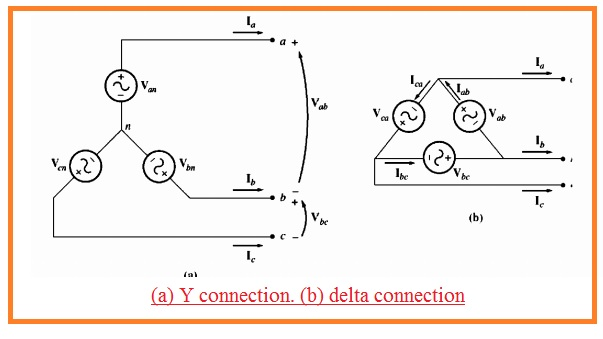• The voltage and current in the resultant phase known as phase quantity and the current flowing in the line and voltage about the line linked to the generator is called phase quantities.
• The relation among the line and phase quantities in the case of generator relies at the category of connection used either wye or delta.
• These relationships will now be explored for each of the Y and 11 connections

Wye Connection Current and Voltage equation

• The wye configured 3 phase generators having abc phase sequence linked to the resistance as load is seen here.• The equation for phase voltage is given here.• as the load at the output terminal of generators is resistant the current in every phase of the generator will has the same value of the angle in voltages.
• So current in every phase is mentioned here.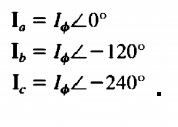• In the case of the diagram, it is certain that the current in any wire is like to the current in the resultant phase.• So in the case of wye connection we have.
• IL=
• The relation between the line voltage and phase voltage is not easy to get.
• So we KVL the value of line to line phase voltage Vab is mentioned here.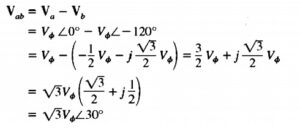• So the equation among the values of line to line voltage and line to neutral voltage for wye configured generator can be seen here.

VLL=√Vθ

• with that, the line voltage has a phase difference from the phase voltage of thirty degrees.
• the phasor diagram in case of wye connected can be seen here.• We can see that the wye connection having a phase sequence of abc in below figure the line voltage are leading the phase voltage through thirty degrees.• In case of wye connection having the acb the phase sequence the line voltage lagging the resultant phase volage through thirty degrees

### Delta Connection Current and Voltages

• the delta configured 3 phase generator linked to the resistance as load can see here.• the equation for phase voltage is written here.• for delta connection, the line-to-line voltage among the 2 lines will be like the voltage for the resultant phase.

VLL=Vθ

• the relation among the current and phase current is not easy to understand so we use KCL### Relationships between Y and Delta connections

• In the below table we can see the wye and delta connection configuration and relation.